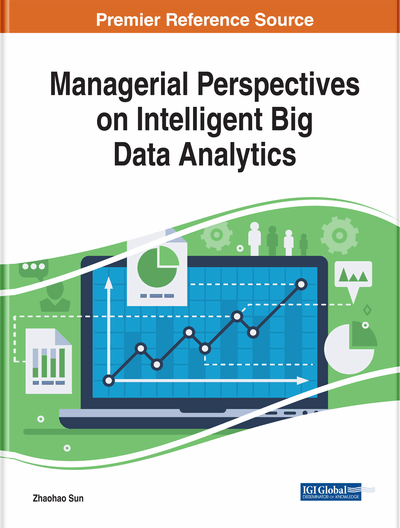# Analysis of Cutting-Edge Regression Algorithms Used for Data Analysis

DOI: 10.4018/978-1-5225-7277-0.ch011
OnDemand:
(Individual Chapters)
Available
\$37.50
No Current Special Offers

## Abstract

Considering the growing applications of big data analytics in the various fields such as healthcare, finance, e-commerce, and web services, it is essential to continuously develop techniques useful for big data. Among various techniques used for big data analytics, regression analysis is very important. In this chapter, an attempt is made to take a detailed look into some of the main regression algorithms and their origin that are used for big data analytics. In this study, some of very famous works related to regression along with some latest research are analyzed. Regression is the process of deducing a predictive model for real-world information based on verified information that is already received. It is used for making predictions, optimizing solutions to complex problems, and understands trends in large and big data analytics. The goal of this study is to promote and facilitate a better understanding of regression algorithms that are in use in the real world for big data analytics.
Chapter Preview
Top

## Background

Before diving into regression and methods to implement it, first it must be mentioned clearly basics and importance of a dataset in big data analytics. Data as the formal definition goes are unprocessed facts, in predictive modeling data has two parts dependent and independent data/variable/feature, goal is to build relation for dependent variable with respect to one or more independent variables. Dependent variables are those variables whose scalar/vector magnitude value depend on independent variables and can be found through some relation among independent variables, on the other hand independent variables are the variables which are not dependent or have any relation with other variables in the dataset. Datasets can be discrete data or continuous data (Pasta, 2009).

Regression algorithms are used to predict continuous variables/data on the basis of one or more independent variables (Rawlings, Pantula, & Dickey, 1998). Attempts at regression date back to the days of the legendary Isaac Newton and Joseph-Louis Lagrange. The crudest attempt at this was interpolation- where, given (n+1) points on a 2-D plane, a polynomial of degree n satisfying all those points can be derived. Then the values at all other points by using this polynomial can be predicted. Unfortunately, such a simple solution cannot be applied in the fabrication of an intelligent device. This method is used for mathematical purposes with purely academic ambition.

The solution relies overly on the training data supplied to it. This is called over-fitting. In Figure1, the red line shows a curve that uses a method like interpolation and exhibits the problem of over-fitting. The green line does not touch each point given, but it is a better predictive model because the total error is less. It shows the difference between a useful regression model and a curve obtained by interpolation which goes through each given data point. At the point labeled 0.9, the approximate error for the red line is much higher than that for the green line. This means, that the green line is a much more accurate predictive model- as mentioned earlier. This is achieved through some of the methods discussed in this study.

## Key Terms in this Chapter

Regression: It is a statistical measure used to determine the strength of the relationship between one dependent variable and a series of other changing variables.

Data Analytics: It is the process of examining data to draw conclusions about the information they contain.

SVM Kernels: SVM algorithms use a set of mathematical functions called kernel which take data as input and transform it into the required form.

Big Data: It is a massive volume of both structured and unstructured data that is difficult to process using traditional techniques.

## Complete Chapter List

Search this Book:
Reset